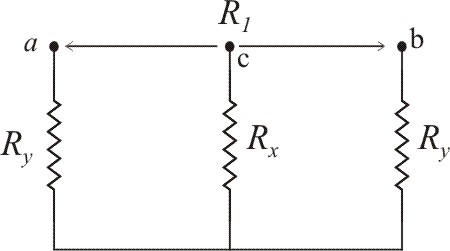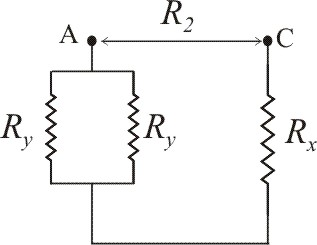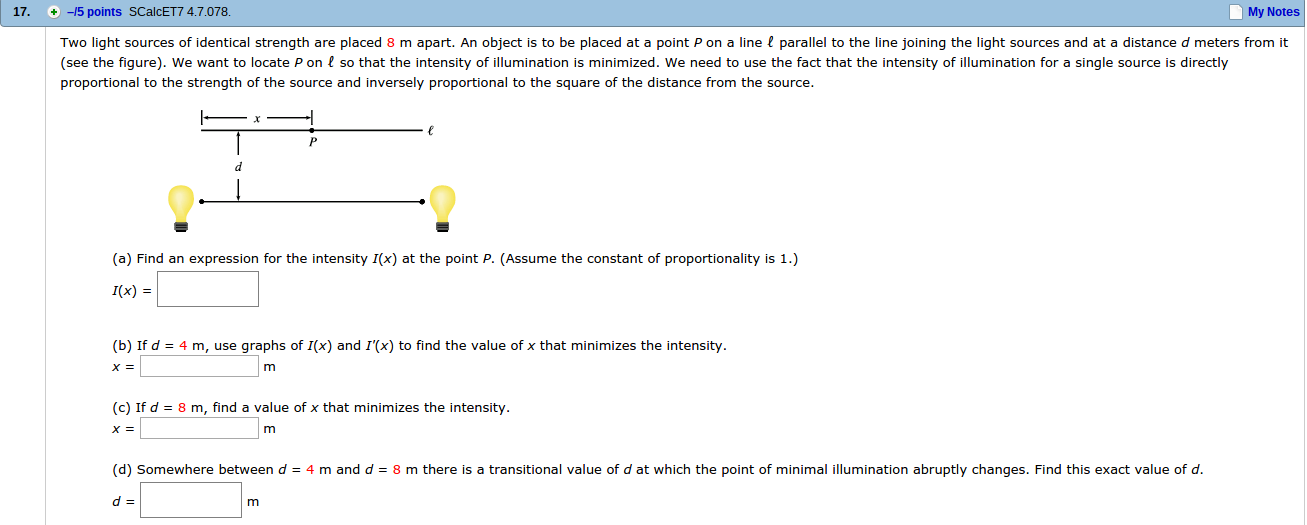Ask question

# The student engineer of a campus radio station wishes to verify the effectivencess of the lightning rod on the antenna mast. The unknown resistance R_x is between points C and E. Point E is a "true ground", but is inaccessible for direct measurement because the stratum in which it is located is several meters below Earth's surface. Two identical rods are driven into the ground at A and B, introducing an unknown resistance R_y. The procedure for finding the unknown resistance R_x is as follows. Measure resistance R_1 between points A and B. Then connect A and B with a heavy conducting wire and measure resistance R_2 between points A and C.Derive a formula for R_x in terms of the observable resistances R_1 and R_2. A satisfactory ground resistance would be R_x<2.0 Ohms. Is the grounding of t# The student engineer of a campus radio station wishes to verify the effectivencess of the lightning rod on the antenna mast. The unknown resistance R_x is between points C and E. Point E is a "true ground", but is inaccessible for direct measurement because the stratum in which it is located is several meters below Earth's surface. Two identical rods are driven into the ground at A and B, introducing an unknown resistance R_y. The procedure for finding the unknown resistance R_x is as follows. Measure resistance R_1 between points A and B. Then connect A and B with a heavy conducting wire and measure resistance R_2 between points A and C.Derive a formula for R_x in terms of the observable resistances R_1 and R_2. A satisfactory ground resistance would be R_x<2.0 Ohms. Is the grounding of t

Question
Otherasked 2021-05-18
The student engineer of a campus radio station wishes to verify the effectivencess of the lightning rod on the antenna mast. The unknown resistance $$\displaystyle{R}_{{x}}$$ is between points C and E. Point E is a "true ground", but is inaccessible for direct measurement because the stratum in which it is located is several meters below Earth's surface. Two identical rods are driven into the ground at A and B, introducing an unknown resistance $$\displaystyle{R}_{{y}}$$. The procedure for finding the unknown resistance $$\displaystyle{R}_{{x}}$$ is as follows. Measure resistance $$\displaystyle{R}_{{1}}$$ between points A and B. Then connect A and B with a heavy conducting wire and measure resistance $$\displaystyle{R}_{{2}}$$ between points A and C.Derive a formula for $$\displaystyle{R}_{{x}}$$ in terms of the observable resistances $$\displaystyle{R}_{{1}}$$ and $$\displaystyle{R}_{{2}}$$. A satisfactory ground resistance would be $$\displaystyle{R}_{{x}}{<}{2.0}$$</span> Ohms. Is the grounding of the station adequate if measurments give $$\displaystyle{R}_{{1}}={13}{O}{h}{m}{s}$$ and R_2=6.0 Ohms?

## Answers (1)2021-05-20

a) Two identical rods are driven into the ground at A and B, introducing an unknown resistance $$\displaystyle{R}_{{y}}$$. The procedure for finding the unknown resistance $$\displaystyle{R}_{{x}}$$ is as follows: measure resistance $$\displaystyle{R}_{{1}}$$ between points A and B. Then connect A and B with a heavy conducting wire, and measure resistance $$\displaystyle{R}_{{2}}$$ between points A and C. This shown in below figure.The equivalent resistance of series resistors $$\displaystyle{R}_{{y}}$$ and $$\displaystyle{R}_{{y}}$$ is
$$\displaystyle{R}_{{1}}={R}_{{y}}+{R}_{{y}}$$
$$\displaystyle={2}{R}_{{y}}$$From the above circuit $$\displaystyle{R}_{{y}}$$ and $$\displaystyle{R}_{{y}}$$ are connected in parallel. The equivalent resistance is
$$\displaystyle{R}_{{{e}{q}}}={\left[{\frac{{{1}}}{{{R}_{{y}}}}}+{\frac{{{1}}}{{{R}_{{y}}}}}\right]}^{{-{1}}}$$
$$\displaystyle={\frac{{{R}_{{y}}}}{{{2}}}}$$
And, $$\displaystyle{R}_{{{e}{q}}}$$ and $$\displaystyle{R}_{{x}}$$ are connected in series. Then the equivalent resistance is
$$\displaystyle{R}_{{2}}={R}_{{{e}{q}}}+{R}_{{x}}$$
$$\displaystyle={\frac{{{R}_{{y}}}}{{{2}}}}+{R}_{{x}}$$
From (1),
$$\displaystyle{R}_{{y}}={\frac{{{R}_{{1}}}}{{{2}}}}$$
Substitute this value in equation, we get
$$\displaystyle\therefore{R}_{{2}}={\frac{{{1}}}{{{2}}}}{\left({\frac{{{R}_{{1}}}}{{{2}}}}\right)}+{R}_{{x}}$$
$$\displaystyle={\frac{{{R}_{{1}}}}{{{4}}}}+{R}_{{x}}$$
$$\displaystyle\therefore{R}_{{x}}={R}_{{2}}-{\frac{{{R}_{{1}}}}{{{4}}}}$$
b) If the measurement give $$\displaystyle{R}_{{1}}={13}$$ Ohm and $$\displaystyle{R}_{{2}}={6.0}$$ Ohm
Then
$$\displaystyle{R}_{{x}}={R}_{{2}}-{\frac{{{R}_{{1}}}}{{{4}}}}$$
$$\displaystyle={\left({6.0}\ {O}{h}{m}\right)}-{\left({\frac{{{13}\ {O}{h}{m}}}{{{4}}}}\right)}$$
$$\displaystyle={2.75}$$ Ohm
$$\displaystyle\approx{2.8}$$ Ohm
Here satisfactory ground resistance would be $$\displaystyle{R}_{{x}}{<}{2.0}$$ Ohm. So $$\displaystyle{R}_{{x}}$$ is exceeds the limit of 2.0 Ohm
Therefore, the antenna is not adequate grounded.

### Relevant Questionsasked 2021-03-29
Two stationary point charges +3 nC and + 2nC are separated bya distance of 50cm. An electron is released from rest at a pointmidway between the two charges and moves along the line connectingthe two charges. What is the speed of the electron when it is 10cmfrom +3nC charge?
Besides the hints I'd like to ask you to give me numericalsolution so I can verify my answer later on. It would be nice ifyou could write it out, but a numerical anser would be fine alongwith the hint how to get there.asked 2021-05-05

A random sample of $$n_1 = 14$$ winter days in Denver gave a sample mean pollution index $$x_1 = 43$$.
Previous studies show that $$\sigma_1 = 19$$.
For Englewood (a suburb of Denver), a random sample of $$n_2 = 12$$ winter days gave a sample mean pollution index of $$x_2 = 37$$.
Previous studies show that $$\sigma_2 = 13$$.
Assume the pollution index is normally distributed in both Englewood and Denver.
(a) State the null and alternate hypotheses.
$$H_0:\mu_1=\mu_2.\mu_1>\mu_2$$
$$H_0:\mu_1<\mu_2.\mu_1=\mu_2$$
$$H_0:\mu_1=\mu_2.\mu_1<\mu_2$$
$$H_0:\mu_1=\mu_2.\mu_1\neq\mu_2$$
(b) What sampling distribution will you use? What assumptions are you making? NKS The Student's t. We assume that both population distributions are approximately normal with known standard deviations.
The standard normal. We assume that both population distributions are approximately normal with unknown standard deviations.
The standard normal. We assume that both population distributions are approximately normal with known standard deviations.
The Student's t. We assume that both population distributions are approximately normal with unknown standard deviations.
(c) What is the value of the sample test statistic? Compute the corresponding z or t value as appropriate.
(Test the difference $$\mu_1 - \mu_2$$. Round your answer to two decimal places.) NKS (d) Find (or estimate) the P-value. (Round your answer to four decimal places.)
(e) Based on your answers in parts (i)−(iii), will you reject or fail to reject the null hypothesis? Are the data statistically significant at level \alpha?
At the $$\alpha = 0.01$$ level, we fail to reject the null hypothesis and conclude the data are not statistically significant.
At the $$\alpha = 0.01$$ level, we reject the null hypothesis and conclude the data are statistically significant.
At the $$\alpha = 0.01$$ level, we fail to reject the null hypothesis and conclude the data are statistically significant.
At the $$\alpha = 0.01$$ level, we reject the null hypothesis and conclude the data are not statistically significant.
(f) Interpret your conclusion in the context of the application.
Reject the null hypothesis, there is insufficient evidence that there is a difference in mean pollution index for Englewood and Denver.
Reject the null hypothesis, there is sufficient evidence that there is a difference in mean pollution index for Englewood and Denver.
Fail to reject the null hypothesis, there is insufficient evidence that there is a difference in mean pollution index for Englewood and Denver.
Fail to reject the null hypothesis, there is sufficient evidence that there is a difference in mean pollution index for Englewood and Denver. (g) Find a 99% confidence interval for
$$\mu_1 - \mu_2$$.
(Round your answers to two decimal places.)
lower limit
upper limit
(h) Explain the meaning of the confidence interval in the context of the problem.
Because the interval contains only positive numbers, this indicates that at the 99% confidence level, the mean population pollution index for Englewood is greater than that of Denver.
Because the interval contains both positive and negative numbers, this indicates that at the 99% confidence level, we can not say that the mean population pollution index for Englewood is different than that of Denver.
Because the interval contains both positive and negative numbers, this indicates that at the 99% confidence level, the mean population pollution index for Englewood is greater than that of Denver.
Because the interval contains only negative numbers, this indicates that at the 99% confidence level, the mean population pollution index for Englewood is less than that of Denver.asked 2021-04-25
A wagon with two boxes of Gold, having total mass 300 kg, is cutloose from the hoses by an outlaw when the wagon is at rest 50m upa 6.0 degree slope. The outlaw plans to have the wagon roll downthe slope and across the level ground, and then fall into thecanyon where his confederates wait. But in a tree 40m from thecanyon edge wait the Lone Ranger (mass 75.0kg) and Tonto (mass60.0kg). They drop vertically into the wagon as it passes beneaththem. a) if they require 5.0 s to grab the gold and jump out, willthey make it before the wagon goes over the edge? b) When the twoheroes drop into the wagon, is the kinetic energy of the system ofthe heroes plus the wagon conserved? If not, does it increase ordecrease and by how much?asked 2021-05-05
The bulk density of soil is defined as the mass of dry solidsper unit bulk volume. A high bulk density implies a compact soilwith few pores. Bulk density is an important factor in influencing root development, seedling emergence, and aeration. Let X denotethe bulk density of Pima clay loam. Studies show that X is normally distributed with $$\displaystyle\mu={1.5}$$ and $$\displaystyle\sigma={0.2}\frac{{g}}{{c}}{m}^{{3}}$$.
(a) What is thedensity for X? Sketch a graph of the density function. Indicate onthis graph the probability that X lies between 1.1 and 1.9. Findthis probability.
(b) Find the probability that arandomly selected sample of Pima clay loam will have bulk densityless than $$\displaystyle{0.9}\frac{{g}}{{c}}{m}^{{3}}$$.
(c) Would you be surprised if a randomly selected sample of this type of soil has a bulkdensity in excess of $$\displaystyle{2.0}\frac{{g}}{{c}}{m}^{{3}}$$? Explain, based on theprobability of this occurring.
(d) What point has the property that only 10% of the soil samples have bulk density this high orhigher?
(e) What is the moment generating function for X?asked 2021-05-16
Consider the curves in the first quadrant that have equationsy=Aexp(7x), where A is a positive constant. Different valuesof A give different curves. The curves form a family,F. Let P=(6,6). Let C be the number of the family Fthat goes through P.
A. Let y=f(x) be the equation of C. Find f(x).
B. Find the slope at P of the tangent to C.
C. A curve D is a perpendicular to C at P. What is the slope of thetangent to D at the point P?
D. Give a formula g(y) for the slope at (x,y) of the member of Fthat goes through (x,y). The formula should not involve A orx.
E. A curve which at each of its points is perpendicular to themember of the family F that goes through that point is called anorthogonal trajectory of F. Each orthogonal trajectory to Fsatisfies the differential equation dy/dx = -1/g(y), where g(y) isthe answer to part D.
Find a function of h(y) such that x=h(y) is the equation of theorthogonal trajectory to F that passes through the point P.asked 2021-02-10
Two light sources of identical strength are placed 8 m apart. An object is to be placed at a point P on a line ? parallel to the line joining the light sources and at a distance d meters from it (see the figure). We want to locate P on ? so that the intensity of illumination is minimized. We need to use the fact that the intensity of illumination for a single source is directly proportional to the strength of the source and inversely proportional to the square of the distance from the source.asked 2021-03-12
A 75.0-kg man steps off a platform 3.10 m above the ground. Hekeeps his legs straight as he falls, but at the moment his feettouch the ground his knees begin to bend, and, treated as aparticle, he moves an additional 0.60 m before coming torest.
a) what is the speed at the instant his feet touch theground?
b) treating him as a particle, what is his acceleration(magnitude and direction) as he slows down, if the acceleration isassumed to be constant?
c) draw his free-body diagram (see section 4.6). in termsof forces on the diagram, what is the net force on him? usenewton's laws and the results of part (b) to calculate the averageforce his feet exert on the ground while he slows down. expressthis force in newtons and also as a multiple of his weight.asked 2021-05-09
The dominant form of drag experienced by vehicles (bikes, cars,planes, etc.) at operating speeds is called form drag. Itincreases quadratically with velocity (essentially because theamount of air you run into increase with v and so does the amount of force you must exert on each small volume of air). Thus
$$\displaystyle{F}_{{{d}{r}{u}{g}}}={C}_{{d}}{A}{v}^{{2}}$$
where A is the cross-sectional area of the vehicle and $$\displaystyle{C}_{{d}}$$ is called the coefficient of drag.
Part A:
Consider a vehicle moving with constant velocity $$\displaystyle\vec{{{v}}}$$. Find the power dissipated by form drag.
Express your answer in terms of $$\displaystyle{C}_{{d}},{A},$$ and speed v.
Part B:
A certain car has an engine that provides a maximum power $$\displaystyle{P}_{{0}}$$. Suppose that the maximum speed of thee car, $$\displaystyle{v}_{{0}}$$, is limited by a drag force proportional to the square of the speed (as in the previous part). The car engine is now modified, so that the new power $$\displaystyle{P}_{{1}}$$ is 10 percent greater than the original power ($$\displaystyle{P}_{{1}}={110}\%{P}_{{0}}$$).
Assume the following:
The top speed is limited by air drag.
The magnitude of the force of air drag at these speeds is proportional to the square of the speed.
By what percentage, $$\displaystyle{\frac{{{v}_{{1}}-{v}_{{0}}}}{{{v}_{{0}}}}}$$, is the top speed of the car increased?
Express the percent increase in top speed numerically to two significant figures.asked 2021-04-18
A 2.0-kg projectile is fired with initial velocity components $$\displaystyle{v}_{{{0}{x}}}={30}$$ m/s and $$\displaystyle{v}_{{{0}{y}}}={40}$$ m/s from a point on the earth's surface. Neglect any effects due to air resistance. What is the kinetic energy of the projectile when it reaches the highest point in its trajectory? How much work was done in firing the projectile?asked 2021-05-20
Assume that a ball of charged particles has a uniformly distributednegative charge density except for a narrow radial tunnel throughits center, from the surface on one side to the surface on the opposite side. Also assume that we can position a proton any where along the tunnel or outside the ball. Let $$\displaystyle{F}_{{R}}$$ be the magnitude of the electrostatic force on the proton when it islocated at the ball's surface, at radius R. As a multiple ofR, how far from the surface is there a point where the forcemagnitude is 0.44FR if we move the proton(a) away from the ball and (b) into the tunnel?
...